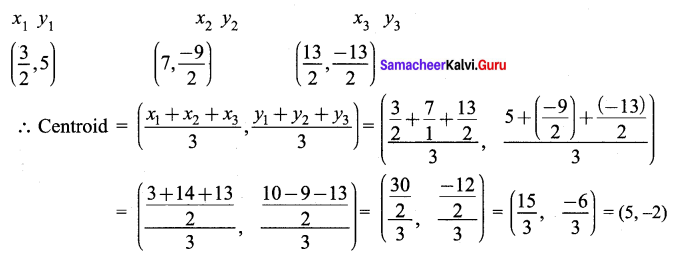# Samacheer Kalvi 9th Maths Solutions Chapter 5 Coordinate Geometry Ex 5.5

## Tamilnadu Samacheer Kalvi 9th Maths Solutions Chapter 5 Coordinate Geometry Ex 5.5

Question 1.
Find the centroid of the triangle whose vertices are
(i) (2, -4 ), (-3, -7) and (7, 2)
(ii) (-5, -5), (1, -4) and (-4, -2)
Solution: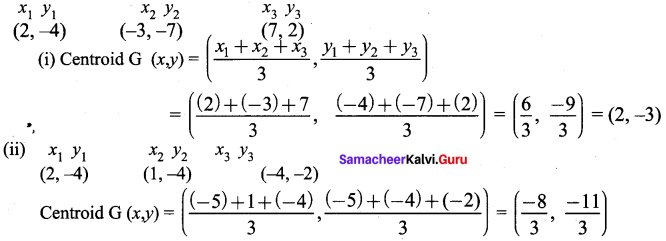Question 2.
If the centroid of a triangle is at (4,-2) and two of its vertices are (3, -2) and (5, 2) then find the third vertex of the triangle.
Solution:
Centroid G (x, y) = (4, -2)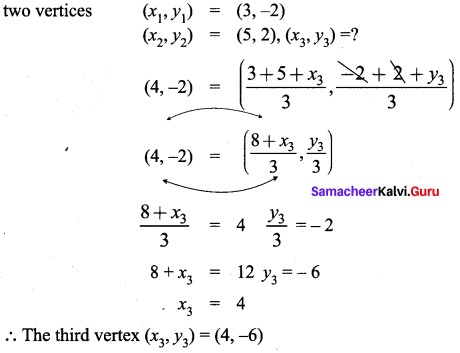Question 3.
Find the length of median through Aof a triangle whose vertices are A(-1, 3), B(1, -1) and C(5, 1).
Solution: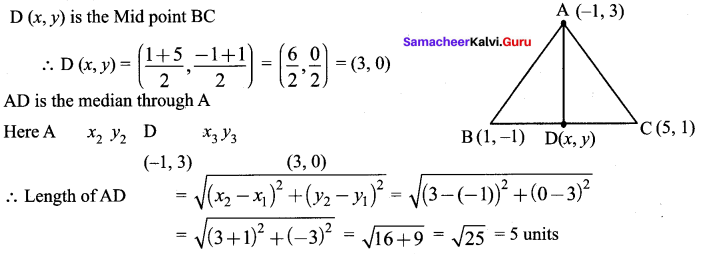Question 4.
The vertices of a triangle are (1, 2), (h, -3) and (-4, k). If the centroid of the triangle is at the point (5, -1) then find the value of $$\sqrt{(h+k)^{2}+(h+3 k)^{2}}$$
Solution: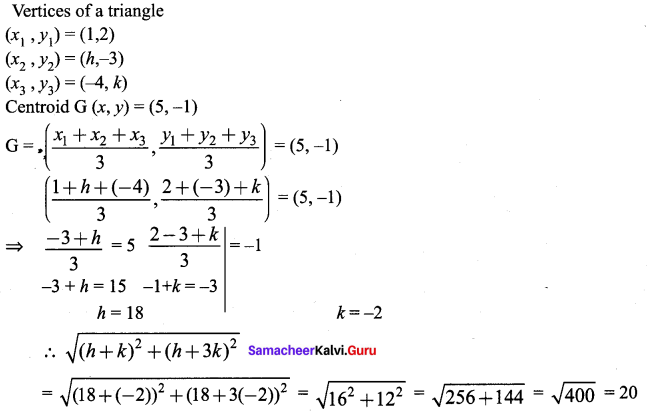Question 5.
Orthocentre and centroid of a triangle are A(-3, 5) and B(3, 3) respectively. If C is the circumcentre and AC is the diameter of this circle, then find the radius of the circle.
Solution: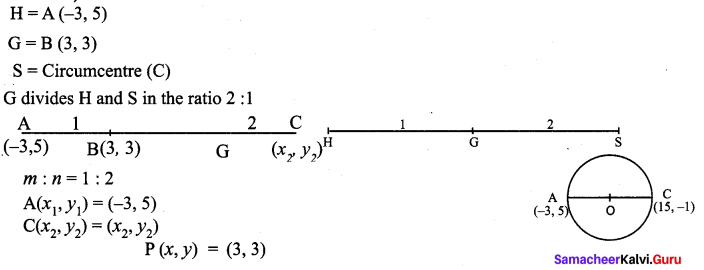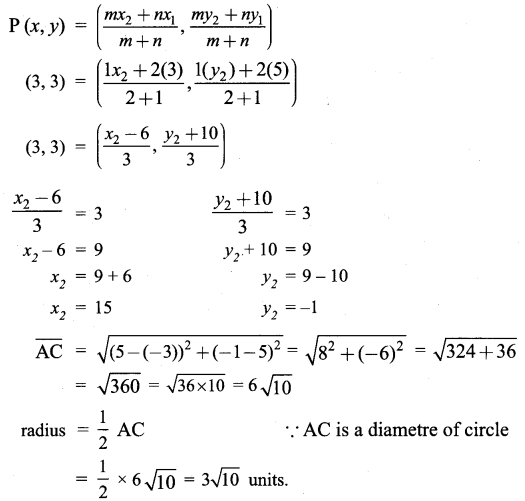Question 6.
ABC is a triangle whose vertices are A(3, 4), B(-2, -1) and C(5, 3). If G is the centroid and BDCG is a parallelogram then find the coordinates of the vertex D.
Solution: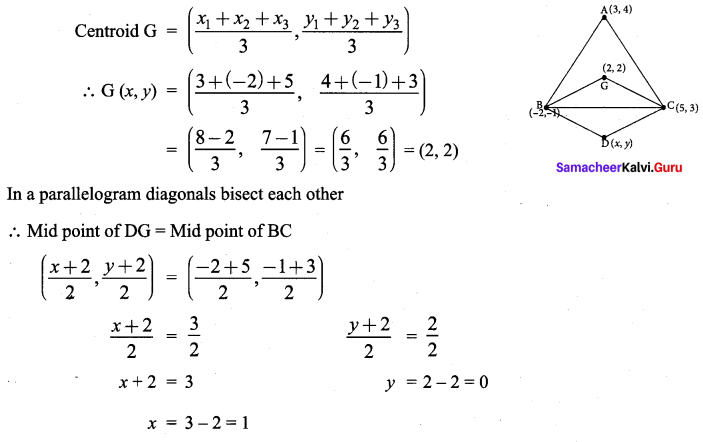∴ The co-ordinates of the vertex D(x, y) = (1, 0)

Question 7.
If   and  are mid points of the sides of a triangle, then find the centroid of the triangle.
Solution:
“The centroid of the triangle obtained by joining the mid points of the sides of a triangle is the same as the centroid of the original triangle.”
∴ The mid points of the sides of the triangle are given as